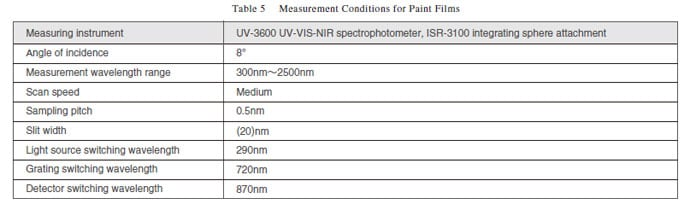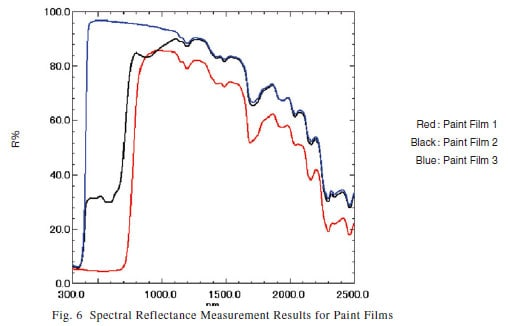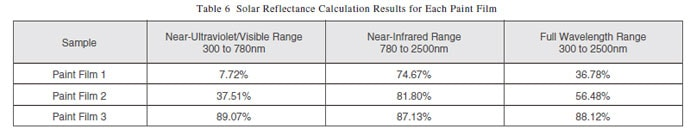# Measuring Solar Transmittance and Solar Reflectance, Part 2

The previous issue, UV Talk Letter Vol. 5, introduced measurement methods for flat glass, in compliance with JIS R3106 "Testing method on transmittance, reflectance and emittance of flat glasses and evaluation of solar heat gain coefficient." This issue introduces measurement methods for films for glazing used in construction according to JIS A5759 "Adhesive films for glazings," and methods for paint film according to JIS K5602 "Determination of reflectance of solar radiation by paint film."

## 1. Evaluation of the Optical Performance of Films for Glazing Used in Construction, Conforming to JIS A5759

JIS A5759 prescribes 87 % max. visible light transmittance, 5.9 W/m2K max. heat transfer coefficient, and 3 % max. ultraviolet transmittance for films applied to glazing used in building windows or entrances. The shading coefficient must match the performance, as shown in Table 1. Table 2 provides a description and equation for each item.

JIS A5759 prescribes four test items for optical performance: visible light transmittance, shading coefficient, heat transfer coefficient, and ultraviolet transmittance. However, determining the shading coefficient requires the corrected emittance values for the indoor surface and outdoor surface converted from the normal reflectance, in addition to the solar transmittance, solar reflectance, and normal emittance. In addition, the corrected emittance values for the indoor and outdoor surfaces converted from the normal emittance are required to calculate the heat transfer coefficient.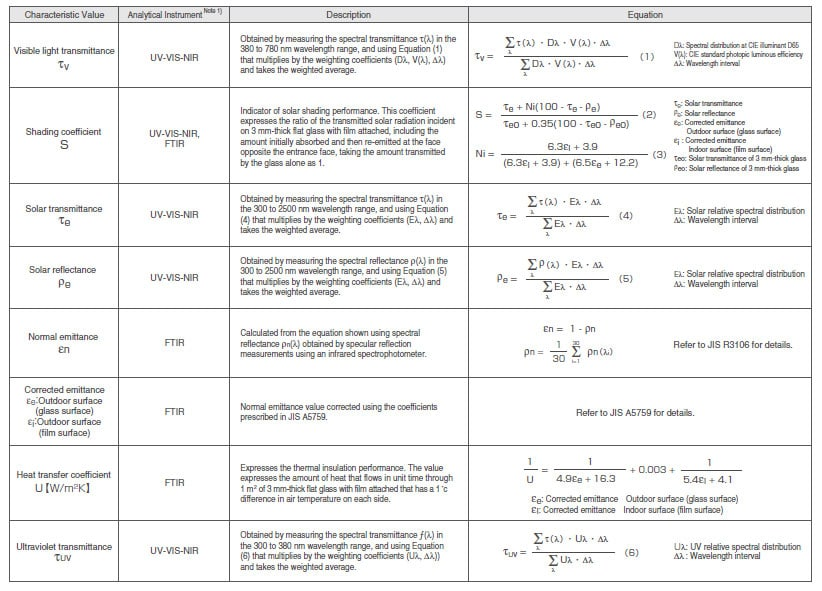Note 1) UV-VIS-NIR = Ultraviolet-Visible-Near Infrared Spectrophotometer; FTIR = Fourier Transform Infrared Spectrophotometer　 The weighting coefficients are described in JIS A5759.

## 2. Examples of Film Measurements

The spectral transmittance is obtained by measuring the transmittance of 3 mm-thick glass with film attached to one face. For these tests, we determined the visible light transmittance, UV transmittance, solar transmittance, solar reflectance, and shading coefficients for four types of film adhered to glass. The measurements were performed on light incident from the glass surface using a UV-VIS-NIR spectrophotometer with an integrating sphere accessory under the conditions shown in Table 3. Fig. 1 shows the transmission spectra in the ultraviolet, visible, and near-infrared regions. Fig. 2 shows the reflection spectra. Data measured by a Fourier transform infrared spectrophotometer was used to calculate the shading coefficients, but details of the measurement method and conditions are omitted here. Solar transmittance measurement software was used to calculate the performance test items. This software supports the calculation of visible light transmittance, UV transmittance, solar transmittance, and solar reflectance for flat glass according to JIS R3106. For these tests, the calculations prescribed in JIS A5759 were performed in the user-defined mode by entering the weighting coefficients. Fig. 3 shows a sample screen to set the calculation items for the solar transmittance measurement software. Fig. 4 shows sample calculated results for the visible light transmittance, UV transmittance, and solar transmittance.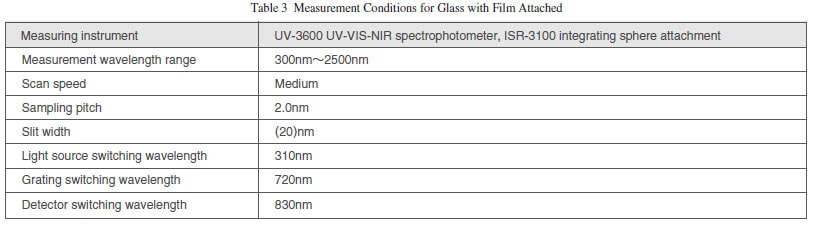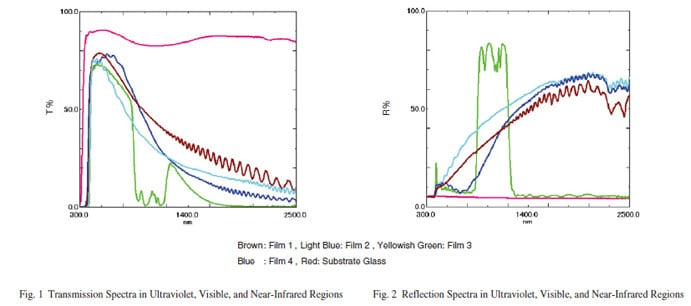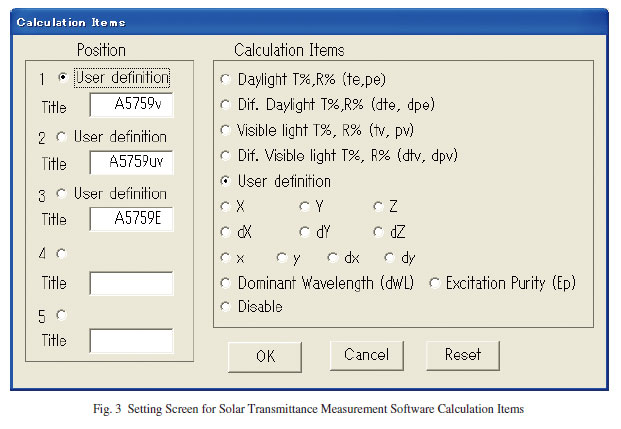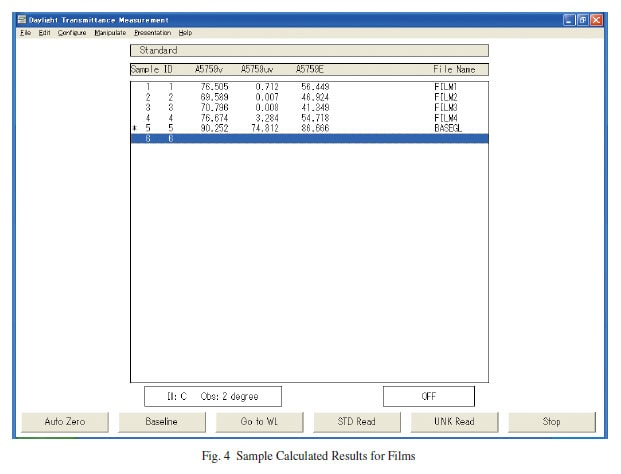Table 4 shows the calculated results for each sample. Film 1 exhibits a high value for solar transmittance. Films 2 and 3 allow almost no transmittance of ultraviolet. Film 4 offers the best transmittance of visible light and ultraviolet and exhibits the highest shading coefficient value.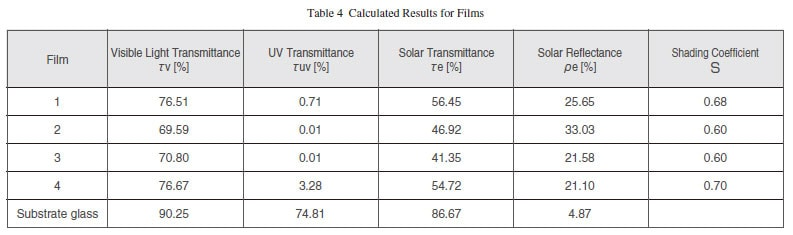## 3. Determination of Solar Reflectance of Paint Film According to JIS K5602

Solar reflectance measurements in accordance with JIS K5602 use the integrating sphere accessory, 300 to 2500 nm wavelength measurement range, and an angle of incidence of light onto the sample not exceeding 15 degrees. The specular reflectance of the sample is determined at each wavelength, based on 100 % spectral reflectance for a standard white sheet calibrated by a public organization. The solar reflectance is obtained using Equation (7) to multiply by the weighting coefficient for the spectral irradiance distribution of standard sunlight and take the weighted average. The solar reflectance is determined individually in three wavelength ranges: near-ultraviolet/visible (300 to 780 nm), near-infrared (780 to 2500 nm), and full wavelength (300 to 2500 nm).

## 4. Examples of Paint Film Measurements

Spectral reflectance measurements were performed on three types of paint applied to contrast ratio test paper (Paint Films 1, 2, 3). Measurements were made using a UV-3600 UV-VIS-NIR spectrophotometer with an ISR-3100 integrating sphere attachment under the conditions listed in Table 5. The sample was positioned against the integrating sphere, as shown in Fig. 5. The measured results are shown in Fig. 6. Paint Film 1 has lower reflectance than the other two paint films across the full wavelength range. Paint Film 2 offers equivalent reflectance to Paint Film 3 in the near-infrared range above 1200 nm but has lower reflectance than Paint Film 3 in the near-infrared to visible range below 1200 nm. Paint Film 3 offers the highest reflectance across the full wavelength range.

The solar reflectance of each paint film was then calculated using the measured spectral reflectance values and Equation (7). Table 6 shows the solar reflectance in each wavelength range. An Excel macro developed to calculate the solar reflectance of paint films was used to perform these calculations in accordance with JIS K5602. This macro reads the measured spectral reflectance data and automatically calculates the solar reflectance in each wavelength range using Excel® Note 2). It also displays the spectral reflectance spectra. Note 2) Excel is a registered trademark of Microsoft Corporation.